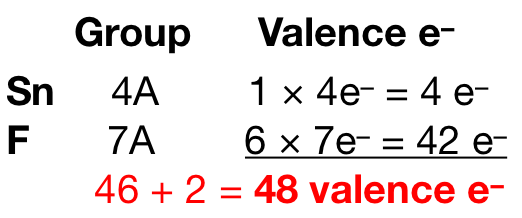Problem: Draw the Lewis structures for each of the following ions or molecules.[SnF6]2-

FREE Expert Solution
82% (164 ratings)
FREE Expert Solution

Before drawing the Lewis structure, we first need to determine which between tin Sn and fluorine F will be the central atom. Tin (EN = 1.96) is less electronegative than fluorine (EN = 3.98) so we designate it as the central atom.

Next, we need to determine the total number of valence electrons present in the molecule. We add 2 electrons to the initial count since the molecule has a charge of –2:Fluorine prefers to form one bond. The Lewis structure of the molecule would look like this:

82% (164 ratings)
Problem Details

Draw the Lewis structures for each of the following ions or molecules.

[SnF6]2-